# Bounding the chromatic number of triangle-free graphs with fixed maximum degree

 Importance: Medium ✭✭
 Author(s): Kostochka, Alexandr V. Reed, Bruce A.
 Subject: Graph Theory » Coloring » » Vertex coloring
 Keywords: chromatic number girth maximum degree triangle free
 Posted by: Andrew King on: April 17th, 2009
Conjecture   A triangle-free graph with maximum degreehas chromatic number at most.

This conjecture is a special case of Reed's,, and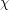conjecture, which posits that for any graph,, where,, andare the clique number, maximum degree, and chromatic number of the graph respectively. Reed's conjecture is very easy to prove for complements of triangle-free graphs, but the triangle-free case seems challenging and interesting in its own right.

This conjecture is very much true for large values of; Johansson proved that triangle-free graphs have chromatic number at most. Surprisingly, the question appears to be open for every value ofgreater than four, up until Johansson's result implies the conjecture.

Kostochka previously proved that the chromatic number of a triangle-free graph is at most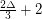, and he proved that for every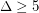there is a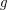for which a graph of girthhas chromatic number at most. Specifically, he showed that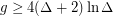is sufficient. In [K] he posed the general problem: "To find the best upper estimate for the chromatic number of the graph in terms of the maximal degree and density or girth."

The conjecture is implied by Brooks' Theorem for. The three smallest open values ofoffer natural entry points to this problem. The easiest seems to be:

Problem   Does there exist a-chromatic triangle-free graph of maximum degree 6?

Perhaps looking at graphs of girth at least five would also be a good starting point.

## Bibliography

[K] Kostochka, A. V., Degree, girth and chromatic number. Combinatorics (Proc. Fifth Hungarian Colloq., Keszthely, 1976), Vol. II, pp. 679--696, Colloq. Math. Soc. János Bolyai, 18, North-Holland, Amsterdam-New York, 1978.

*[R] Reed, B.A.,, and, J. Graph Theory 27 (1998) 177-212.

* indicates original appearance(s) of problem.